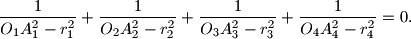### IMO Shortlist 2011 problem G2

Kvaliteta:
Avg: 0,0
Težina:
Avg: 6,0
Let$A_1A_2A_3A_4$ be a non-cyclic quadrilateral. Let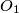$O_1$ and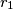$r_1$ be the circumcentre and the circumradius of the triangle$A_2A_3A_4$. Define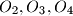$O_2,O_3,O_4$ and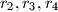$r_2,r_3,r_4$ in a similar way. Prove that